Hostname: page-component-7d684dbfc8-lxvtp Total loading time: 0 Render date: 2023-09-29T21:38:08.224Z Has data issue: false Feature Flags: { "corePageComponentGetUserInfoFromSharedSession": true, "coreDisableEcommerce": false, "coreDisableSocialShare": false, "coreDisableEcommerceForArticlePurchase": false, "coreDisableEcommerceForBookPurchase": false, "coreDisableEcommerceForElementPurchase": false, "coreUseNewShare": true, "useRatesEcommerce": true } hasContentIssue false

# Interlacing polynomials and the veronese construction for rational formal power series

Published online by Cambridge University Press:  30 January 2019

## Abstract

Fixing a positive integer r and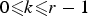$0 \les k \les r-1$, define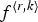$f^{\langle r,k \rangle }$ for every formal power series f as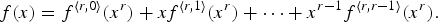$f(x) = f^{\langle r,0 \rangle }(x^r)+xf^{\langle r,1 \rangle }(x^r)+ \cdots +x^{r-1}f^{\langle r,r-1 \rangle }(x^r).$ Jochemko recently showed that the polynomial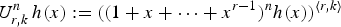$U^{n}_{r,k}\, h(x) := ( (1+x+\cdots +x^{r-1})^{n} h(x) )^{\langle r,k \rangle }$ has only non-positive zeros for any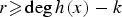$r \ges \deg h(x) -k$ and any positive integer n. As a consequence, Jochemko confirmed a conjecture of Beck and Stapledon on the Ehrhart polynomial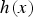$h(x)$ of a lattice polytope of dimension n, which states that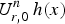$U^{n}_{r,0}\,h(x)$ has only negative, real zeros whenever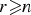$r\ges n$. In this paper, we provide an alternative approach to Beck and Stapledon's conjecture by proving the following general result: if the polynomial sequence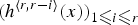$( h^{\langle r,r-i \rangle }(x))_{1\les i \les r}$ is interlacing, so is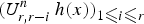$( U^{n}_{r,r-i}\, h(x) )_{1\les i \les r}$. Our result has many other interesting applications. In particular, this enables us to give a new proof of Savage and Visontai's result on the interlacing property of some refinements of the descent generating functions for coloured permutations. Besides, we derive a Carlitz identity for refined coloured permutations.

Type
Research Article

## Access options

Get access to the full version of this content by using one of the access options below. (Log in options will check for institutional or personal access. Content may require purchase if you do not have access.)

## References

1Athanasiadis, C. A.. Edgewise subdivisions. local h-polynomials, and excedances in the wreath product${\open Z}_r{\rm \wr }{\rm {\frak S}}_n$. SIAM J. Discrete Math. 28 (2014), 14791492.CrossRefGoogle Scholar
2Bagno, E. and Biagioli, R.. Colored-descent representations of complex reflection groups$G(r,p,n)$. Israel J. Math. 160 (2007), 317347.CrossRefGoogle Scholar
3Beck, M. and Robins, S.. Computing the continuous discretely. Undergraduate texts in Mathematics 2nd edn (New York: Springer, 2015).CrossRefGoogle Scholar
4Beck, M. and Stapledon, A.. On the log-concavity of Hilbert series of Veronese subrings and Ehrhart series. Math. Z. 264 (2010), 195207.CrossRefGoogle Scholar
5Björner, A.. Some combinatorial and algebraic properties of Coxeter complexes and Tits buildings. Adv. Math. 52 (1984), 173212.CrossRefGoogle Scholar
6Brändén, P.. Unimodality: log-concavity, real-rootedness and beyond, in Handbook of Enumerative Combinatorics. Edited by Miklós Bóna (Boca Raton, FLress: CRC Press, 2015), 437484.CrossRefGoogle Scholar
7Brenti, F. and Welker, V.. f-vectors of barycentric subdivisions. Math. Z. 259 (2008), 849865.CrossRefGoogle Scholar
8Brenti, F. and Welker, V.. The Veronese construction for formal power series and graded algebras. Adv. Appl. Math. 42 (2009), 545556.CrossRefGoogle Scholar
9Brun, M. and Römer, T.. Subdivisions of toric complexes. J. Algebraic Combin. 21 (2005), 423448.CrossRefGoogle Scholar
10Bruns, W. and Herzog, J.. Cohen-Macaulay rings, vol. 39 of Cambridge Studies in Advanced Mathematics (Cambridge: Cambridge University Press, 1993).Google Scholar
11Carlitz, L.. A combinatorial property of q-Eulerian numbers. Am. Math. Monthly 82 (1975), 5154.CrossRefGoogle Scholar
12Chow, C.-O. and Gessel, I. M.. On the descent numbers and major indices for the hyperoctahedral group. Adv. Appl. Math. 38 (2007), 275301.CrossRefGoogle Scholar
13Chow, C.-O. and Mansour, T.. A Carlitz identity for the wreath product${\open Z}_r{\rm \wr }{\rm {\frak S}}_n$. Adv. Appl. Math. 47 (2011), 199215.CrossRefGoogle Scholar
14Dolgachev, I. and Lunts, V.. A character formula for the representation of a Weyl group in the cohomology of the associated toric variety. J. Algebra 168 (1994), 741772.CrossRefGoogle Scholar
15Eisenbud, D., Reeves, A. and Totaro, B.. Initial ideals, Veronese subrings and rates of algebras. Adv. Math. 109 (1994), 168187.CrossRefGoogle Scholar
16Fisk, S.. Polynomials, roots, and interlacing, arXiv:0612833.Google Scholar
17Jochemko, K.. On the real-rootedness of the veronese construction for rational formal power series. Int. Math. Res. Not. 15 (2018), 47804798.CrossRefGoogle Scholar
18Jochemko, K. and Sanyal, R.. Combinatorial positivity of translation-invariant valuations and a discrete hadwiger theorem. J. Eur. Math. Soc. 20 (2018), 21812208.CrossRefGoogle Scholar
19Kubitzke, M. and Welker, V.. Enumerative g-theorems for the Veronese construction for formal power series and graded algebras. Adv. Appl. Math. 49 (2012), 307325.CrossRefGoogle Scholar
20Leander, M.. Compatible polynomials and edgewise subdivisions, arXiv:1605.05287.Google Scholar
21Lin, Z.. On the descent polynomial of signed multipermutations. Proc. Am. Math. Soc. 143 (2015), 36713685.CrossRefGoogle Scholar
22Moynihan, M.. The Flag Descent Algebra and the Colored Eulerian Descent Algebra. Thesis (Ph.D.)–Brandeis University. ProQuest LLC, Ann Arbor, MI (2012).Google Scholar
23Petersen, T. K.. Two-sided Eulerian numbers via balls in boxes. Math. Mag. 86 (2013), 159176.CrossRefGoogle Scholar
24Savage, C. D. and Visontai, M.. The s-Eulerian polynomials have only real roots. Trans. Am. Math. Soc. 367 (2015), 14411466.CrossRefGoogle Scholar
25Stanley, R. P.. The number of faces of a simplicial convex polytope. Adv. Math. 35 (1980), 236238.CrossRefGoogle Scholar
26Stanley, R. P.. Enumerative combinatorics, vol. 49 of Cambridge Studies in Advanced Mathematics, vol. 1 2nd edn (Cambridge: Cambridge University Press, 2012).Google Scholar
27Steingrímsson, E., Permutations statistics of indexed and poset permutations, ProQuest LLC, Ann Arbor, MI, 1992. Thesis (Ph.D.)–Massachusetts Institute of Technology.Google Scholar
28Steingrímsson, E.. Permutation statistics of indexed permutations. Eur. J. Combin. 15 (1994), 187205.CrossRefGoogle Scholar
29Stembridge, J. R.. Eulerian numbers, tableaux, and the Betti numbers of a toric variety. Discrete Math. 99 (1992), 307320.CrossRefGoogle Scholar
30Yang, A. L. and Zhang, P. B.. Mutual interlacing and Eulerian-like polynomials for Weyl groups, arXiv:1401.6273.Google Scholar
31Yang, A. L. and Zhang, P. B.. The Real-rootedness of Eulerian polynomials via the Hermite–Biehler theorem. Discrete Math. Theor. Comput. Sci. Proc. FPSAC 2015, (2015), 465474.Google Scholar
32Zhang, P. B.. On the real-rootedness of the descent polynomials of$(n-2)$-stack sortable permutations. Electron. J. Combin. 22 (2015), P4.12.Google Scholar